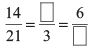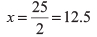Worksheet Question: Ratio & Proportion

# Worksheet Question: Ratio & Proportion - Notes | Study Mathematics (Maths) Class 6 - Class 6

 1 Crore+ students have signed up on EduRev. Have you?

Q.1. Fill in the box :Ans. 2, 9

Q.2. Find the ratio of the following :
(i) 21 hours to 49 hours __________
(ii) 75 cm to 3 m __________
(iii) A dozen to a score __________
(iv) 1 hour to 20 minutes __________
(v) A dozen to a gross __________
Ans.
(i) 21 : 49 = 3 : 7
(ii) 75 : 300 = 1 : 4
(iii) 12 : 20 = 3 : 5
(iv) 60 : 20 = 3 : 1
(v) 12 : 144 = 1 : 12

Q.3. Write True or False :
(i) 2 : 8 :: 4 : 16 (    )
(ii) 500 : 200 :: 150 : 60 (    )
(iii) 50 : 45 :: 30 : 20 (    )
Ans.
(i) True
(ii) True
(iii) False

Q.4. Fill in the blanks so that the numbers are in proportion :
(i) 20, 18, 40, ..............
(ii) ............., 35, 3, 15
(iii) 25, 100, ..........., 160
(iv) 32, ..........., 6, 12
Ans.
(i) 36
(ii) 7
(iii) 40
(iv) 64

Q.5. Find x, if the numbers are in proportion :
(i) 3, 9, 9, x __________
(ii) 25, x, 1 , 4 __________
Ans.
(i) 27
(ii) 100

Q.6. Divide  60  in the ratio 1:2 between Bulbul and Kanika :
(i) Bulbul's share = __________
(ii) Kanika's share = __________
Ans.
(i) 20
(ii) 40

Q.7. Give two equivalent ratios of 6 : 4
Ans. 3 : 2, 12 : 8 (or any other two equivalent ratios)

Q.8. Weight of 80 books in 160 kg. What is the weight of 25 books?
Ans. 50 kg

Q.9. Divide 1200 among A, B and C in the ratios 4 : 5 : 6.
(i) A's share = __________
(ii) B's share = __________
(iii) C's share = __________
In a class of 40 students, 15 like cricket, 20 like football and 5 like both cricket and football. (Now answer question number 10 and 11) :
Ans.
(i) 320
(ii) 400
(iii) 480

Q.10. Find the ratio of number of students who like cricket to those who like football.
Ans. 20 : 25 = 4 : 5

Q.11. Find the ratio of number of students who like football to those who like both.
Ans. 25 : 5 = 5 : 1

Q.12. If 2, 5 and x are in proportion, find x __________ [Hind 2 : 5 :: 5: x]
Ans.Q.13. Find the ratio of 5 days to 2 weeks.
Ans. 5 : 14

Q.14. The cost of one dozen bananas is 60. Find the cost of 8 bananas.
Ans. 40

Q.15. In a class of 48 students, there are 12 girls and rest are boys. Find the ratio of :
(i) Girls to Boys. __________
(ii) Girls to total number of students. __________
Ans.
(i) 12 : 36 = 1 : 3
(ii) 12 : 48 = 1 : 4

The document Worksheet Question: Ratio & Proportion - Notes | Study Mathematics (Maths) Class 6 - Class 6 is a part of the Class 6 Course Mathematics (Maths) Class 6.
All you need of Class 6 at this link: Class 6

## Mathematics (Maths) Class 6

168 videos|282 docs|43 tests
 Use Code STAYHOME200 and get INR 200 additional OFF

## Mathematics (Maths) Class 6

168 videos|282 docs|43 tests

Track your progress, build streaks, highlight & save important lessons and more!

,

,

,

,

,

,

,

,

,

,

,

,

,

,

,

,

,

,

,

,

,

;